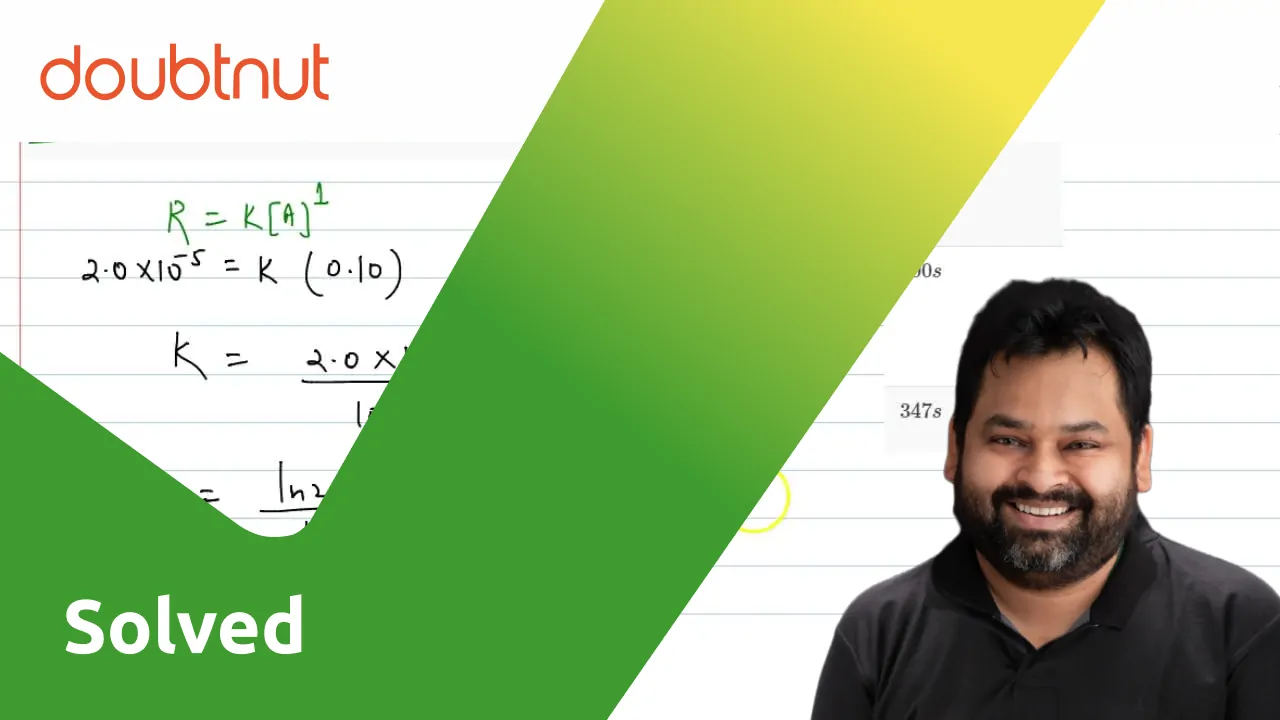Doubtnut App - Get 50 Lakh+ video solutions better than GoogleInstall Now

# For a first-order reaction A→B the reaction rate at reactant concentration of 0.10M is found to be 2.0×10−5molL−1s−1. The half-life period of the reaction is

A

30 s

B

220 s

C

300 s

D

347 s

Text Solution
Verified by Experts

## Given [A]=0.01 M Rate=2.0×10−5molL−1s−1 For a first order reaction Rate=k[A] k=2.0×10−5[0.01]=2×10−3 t1/2=0.6932×10−3=347 sec